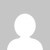# Bayesian network in Python using pgmpy

[wptelegram-join-channel]

## Python Program to Implement the Bayesian network using pgmpy

Exp. No. 7.  Write a program to construct a Bayesian network considering medical data. Use this model to demonstrate the diagnosis of heart patients using a standard Heart Disease Data Set. You can use Java/Python ML library classes/API.

# Theory

A Bayesian network is a directed acyclic graph in which each edge corresponds to a conditional dependency, and each node corresponds to a unique random variable.

Bayesian network consists of two major parts: a directed acyclic graph and a set of conditional probability distributions

• The directed acyclic graph is a set of random variables represented by nodes.
• The conditional probability distribution of a node (random variable) is defined for every possible outcome of the preceding causal node(s).

For illustration, consider the following example. Suppose we attempt to turn on our computer, but the computer does not start (observation/evidence). We would like to know which of the possible causes of computer failure is more likely. In this simplified illustration, we assume only two possible causes of this misfortune: electricity failure and computer malfunction.

The corresponding directed acyclic graph is depicted in below figure.

The goal is to calculate the posterior conditional probability distribution of each of the possible unobserved causes given the observed evidence, i.e. P [Cause | Evidence].

## Data Set:

Title: Heart Disease Databases

The Cleveland database contains 76 attributes, but all published experiments refer to using a subset of 14 of them. In particular, the Cleveland database is the only one that has been used by ML researchers to this date. The “Heartdisease” field refers to the presence of heart disease in the patient. It is integer valued from 0 (no presence) to 4.

Database: 0 1 2 3 4 Total

Cleveland: 164 55 36 35 13 303

Attribute Information:

1. age: age in years
2. sex: sex (1 = male; 0 = female)
3. cp: chest pain type
1. Value 1: typical angina
2. Value 2: atypical angina
3. Value 3: non-anginal pain
4. Value 4: asymptomatic
4. trestbps: resting blood pressure (in mm Hg on admission to the hospital)
5. chol: serum cholestoral in mg/dl
6. fbs: (fasting blood sugar > 120 mg/dl) (1 = true; 0 = false)
7. restecg: resting electrocardiographic results
1. Value 0: normal
2. Value 1: having ST-T wave abnormality (T wave inversions and/or ST elevation or depression of > 0.05 mV)
3. Value 2: showing probable or definite left ventricular hypertrophy by Estes’ criteria
8. thalach: maximum heart rate achieved
9. exang: exercise induced angina (1 = yes; 0 = no)
10. oldpeak = ST depression induced by exercise relative to rest
11. slope: the slope of the peak exercise ST segment
1. Value 1: upsloping
2. Value 2: flat
3. Value 3: downsloping
12. thal: 3 = normal; 6 = fixed defect; 7 = reversable defect
13. Heartdisease: It is integer valued from 0 (no presence) to 4.

## Some instance from the dataset:

### Python Program to Implement and Demonstrate Bayesian network using pgmpy Machine Learning

```import numpy as np
import pandas as pd
import csv
from pgmpy.estimators import MaximumLikelihoodEstimator
from pgmpy.models import BayesianModel
from pgmpy.inference import VariableElimination

heartDisease = heartDisease.replace('?',np.nan)

print('Sample instances from the dataset are given below')

print('\n Attributes and datatypes')
print(heartDisease.dtypes)

model= BayesianModel([('age','heartdisease'),('sex','heartdisease'),('exang','heartdisease'),('cp','heartdisease'),('heartdisease','restecg'),('heartdisease','chol')])
print('\nLearning CPD using Maximum likelihood estimators')
model.fit(heartDisease,estimator=MaximumLikelihoodEstimator)

print('\n Inferencing with Bayesian Network:')
HeartDiseasetest_infer = VariableElimination(model)

print('\n 1. Probability of HeartDisease given evidence= restecg')
q1=HeartDiseasetest_infer.query(variables=['heartdisease'],evidence={'restecg':1})
print(q1)

print('\n 2. Probability of HeartDisease given evidence= cp ')
q2=HeartDiseasetest_infer.query(variables=['heartdisease'],evidence={'cp':2})
print(q2)```

## Summary

This tutorial discusses how to Implement and demonstrate the Bayesian network in Python using pgmpy. If you like the tutorial share it with your friends. Like the Facebook page for regular updates and YouTube channel for video tutorials.

### 4 thoughts on “Bayesian network in Python using pgmpy”

1.Traceback (most recent call last):
File “k:\MACHINE LEARNING LAB\MACHINE LEARNING CASE STUDY\cas.py”, line 19, in
model.fit(heartDisease,estimator=MaximumLikelihoodEstimator)
File “C:\Users\RaKuL\AppData\Local\Packages\PythonSoftwareFoundation.Python.3.9_qbz5n2kfra8p0\LocalCache\local-packages\Python39\site-packages\pgmpy\models\BayesianNetwork.py”, line 535, in fit
_estimator = estimator(
File “C:\Users\RaKuL\AppData\Local\Packages\PythonSoftwareFoundation.Python.3.9_qbz5n2kfra8p0\LocalCache\local-packages\Python39\site-packages\pgmpy\estimators\MLE.py”, line 57, in __init__
super(MaximumLikelihoodEstimator, self).__init__(model, data, **kwargs)
File “C:\Users\RaKuL\AppData\Local\Packages\PythonSoftwareFoundation.Python.3.9_qbz5n2kfra8p0\LocalCache\local-packages\Python39\site-packages\pgmpy\estimators\base.py”, line 209, in __init__
raise ValueError(
ValueError: variable names of the model must be identical to column names in data

1.Hi Rakul,
The dataset contains column name as ‘Heartdisease’ (where ‘H’ is capital) and code for defining the model passing node name as ‘heartdisease’ (where ‘h’ is small). This is why it is throwing an error.

2.The problem with variable names can be fixed by changing ‘sex’ to ‘gender’.

3.error in line no 17